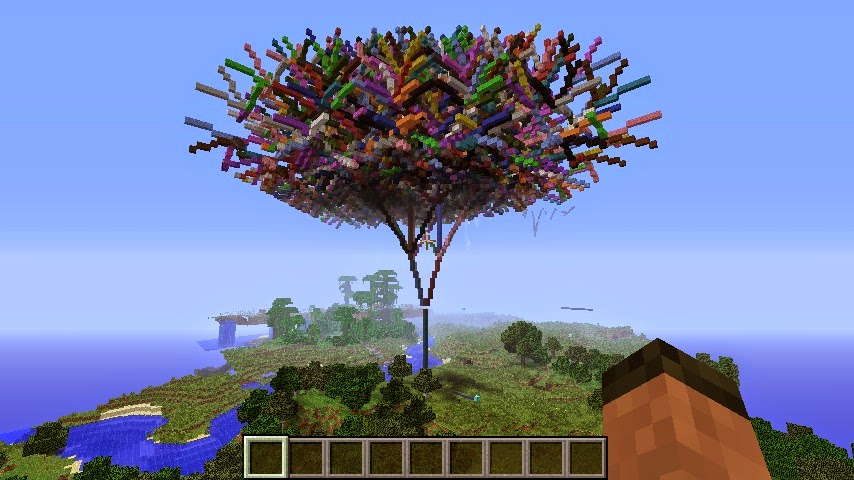# MinecraftTurtle¶

The Minecraft Turtle is a recreation of the classic graphics turtle for Minecraft. The key difference is that you can draw in 3 dimensions not just 2.Example:

```from mcpi.minecraft import Minecraft
from mcpi import block
from minecraftstuff import MinecraftTurtle

mc = minecraft.Minecraft.create()
pos = mc.player.getTilePos()

# create minecraft turtle
steve = MinecraftTurtle(mc, pos)

# draw a pentagon
steve.forward(5)
steve.right(72)
steve.forward(5)
steve.right(72)
steve.forward(5)
steve.right(72)
steve.forward(5)
steve.right(72)
steve.forward(5)
```

## MinecraftTurtle¶

class `minecraftstuff.``MinecraftTurtle`(mc, position=<MagicMock name='mock()' id='139984989718456'>)

MinecraftTurle - a graphics turtle, which can be used to create ‘things’ in Minecraft by controlling its position, angles and direction

Parameters: mc (mcpi.minecraft.Minecraft) – A Minecraft object which is connected to a world. position (mcpi.minecraft.Vec3) – The position where the shape should be created, defaults to `0,0,0`.
`backward`(distance)

move the turtle backward

Parameters: distance (int) – the number of blocks to move.
`down`(angle)

rotate the turtle down

Parameters: angle (float) – the angle in degrees to rotate.
`fly`()

sets the turtle to ‘fly’, i.e. not have to move along the ground.

`forward`(distance)

move the turtle forward

Parameters: distance (int) – the number of blocks to move.
`home`()

reset the turtle’s position

`isdown`()

returns `True` if the pen is down

`left`(angle)

rotate the turtle left

Parameters: angle (float) – the angle in degrees to rotate.
`penblock`(blockId, blockData=0)

set the block the turtle uses as its pen.

Parameters: blockType (int) – The block id. blockData (int) – The block data value, defaults to `0`.
`pendown`()

put the turtles pen down, show it will draw

`penup`()

put the turtles pen up, show it wont draw

`right`(angle)

rotate the turtle right

Parameters: angle (float) – the angle in degrees to rotate.
`setheading`(angle)

Parameters: angle (float) – the angle in degrees.
`setposition`(x, y, z)

set the turtle’s position

Parameters: x (int) – the x position. y (int) – the y position. z (int) – the z position.
`setverticalheading`(angle)

Parameters: angle (float) – the angle in degrees.
`setx`(x)

set the turtle’s x position

Parameters: x (int) – the x position.
`sety`(y)

set the turtle’s y position

Parameters: y (int) – the y position.
`setz`(z)

set the turtle’s z position

Parameters: z (int) – the z position.
`speed`(turtlespeed)

set the turtle’s speed.

Parameters: turtlespeed (int) – `1` - `10`, 1 being the slowest, 10 being the fastest, defaults to `6`. When set to `0` the turtle draws instantaneously.
`up`(angle)

rotate the turtle up

Parameters: angle (float) – the angle in degrees to rotate.
`walk`()

sets the turtle to ‘walk’, i.e. it has to move along the ground.